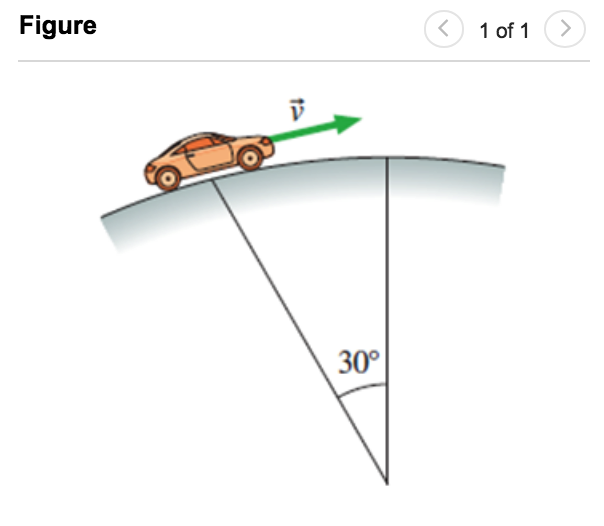# Problem: A 1100 kg car drives at 30 m/s over a circular hill that has a radius of 400 m as shown in (Figure 1). At the point shown in (Figure 1), what is the normal force on the car?

###### FREE Expert Solution

Newton's second law:

$\overline{){\mathbf{F}}{\mathbf{=}}{\mathbf{m}}{\mathbf{a}}}$

Centripetal force:

$\overline{){{\mathbf{F}}}_{{\mathbf{C}}}{\mathbf{=}}\frac{{\mathbf{mv}}^{\mathbf{2}}}{\mathbf{r}}}$

Newton's second law implies that the net force is equal to the centripetal force.

Normal force acts perpendicular to the surface upwards.

mg cos θ is perpendicular to the surface and pulls the cars downwards

Fc acts perpendicular to the surface and pulls the car downwards (towards the center of the circle).

100% (163 ratings)###### Problem Details

A 1100 kg car drives at 30 m/s over a circular hill that has a radius of 400 m as shown in (Figure 1). At the point shown in (Figure 1), what is the normal force on the car?